Скачать презентацию The Logic of Individual Choice The Foundation of

a22cec0aef194ae60a22da2e07b5d72d.ppt

• Количество слайдов: 55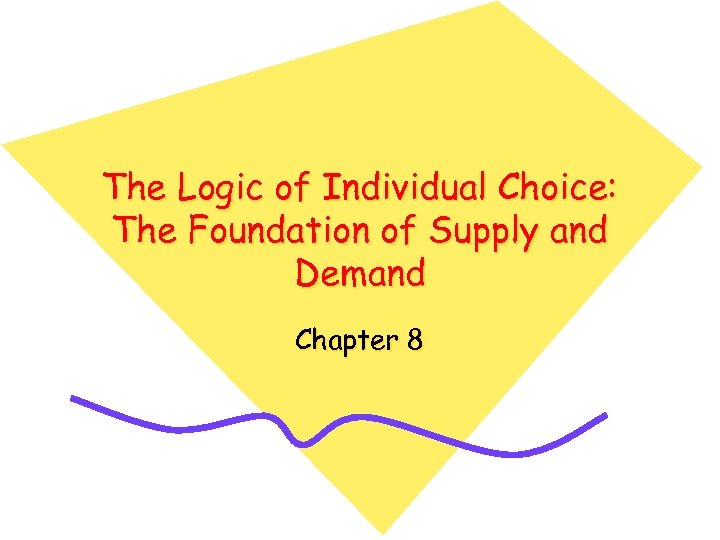The Logic of Individual Choice: The Foundation of Supply and Demand Chapter 8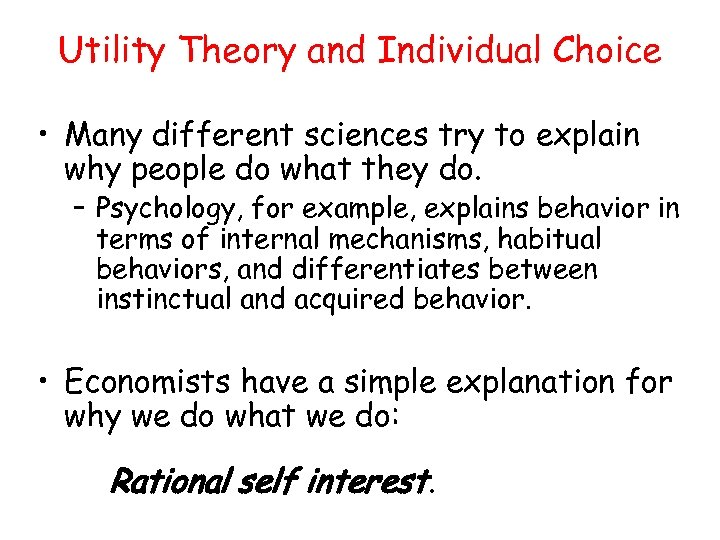Utility Theory and Individual Choice • Many different sciences try to explain why people do what they do. – Psychology, for example, explains behavior in terms of internal mechanisms, habitual behaviors, and differentiates between instinctual and acquired behavior. • Economists have a simple explanation for why we do what we do: Rational self interest.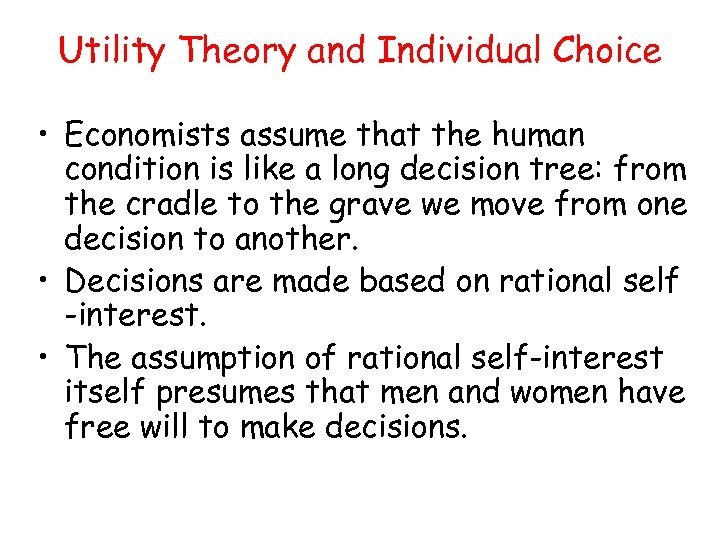Utility Theory and Individual Choice • Economists assume that the human condition is like a long decision tree: from the cradle to the grave we move from one decision to another. • Decisions are made based on rational self -interest. • The assumption of rational self-interest itself presumes that men and women have free will to make decisions.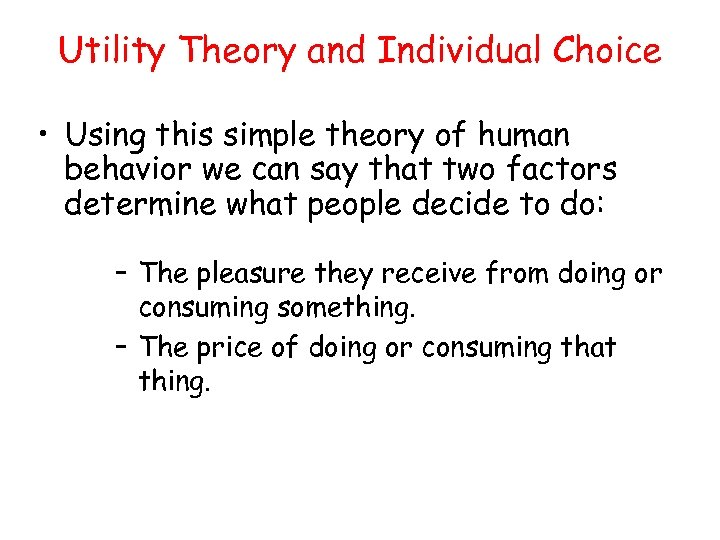Utility Theory and Individual Choice • Using this simple theory of human behavior we can say that two factors determine what people decide to do: – The pleasure they receive from doing or consuming something. – The price of doing or consuming that thing.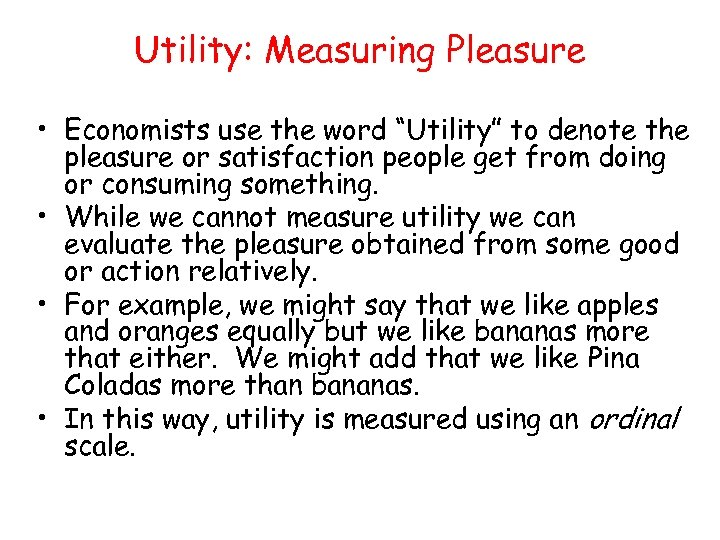Utility: Measuring Pleasure • Economists use the word “Utility” to denote the pleasure or satisfaction people get from doing or consuming something. • While we cannot measure utility we can evaluate the pleasure obtained from some good or action relatively. • For example, we might say that we like apples and oranges equally but we like bananas more that either. We might add that we like Pina Coladas more than bananas. • In this way, utility is measured using an ordinal scale.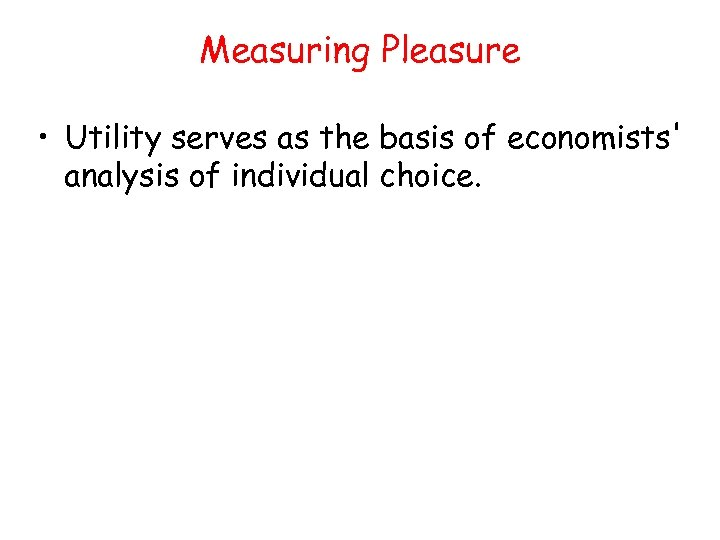Measuring Pleasure • Utility serves as the basis of economists' analysis of individual choice.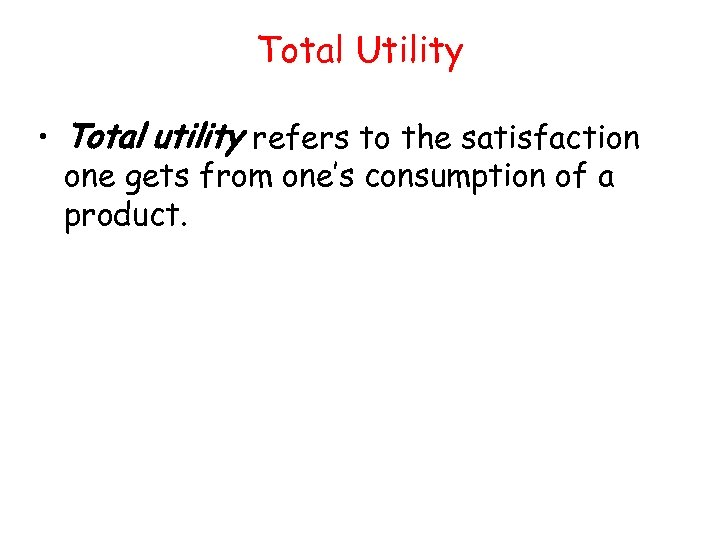Total Utility • Total utility refers to the satisfaction one gets from one’s consumption of a product.Marginal Utility • Marginal utility refers to the satisfaction you get from the consumption of one additional unit of a product above and beyond what you have consumed up to that point.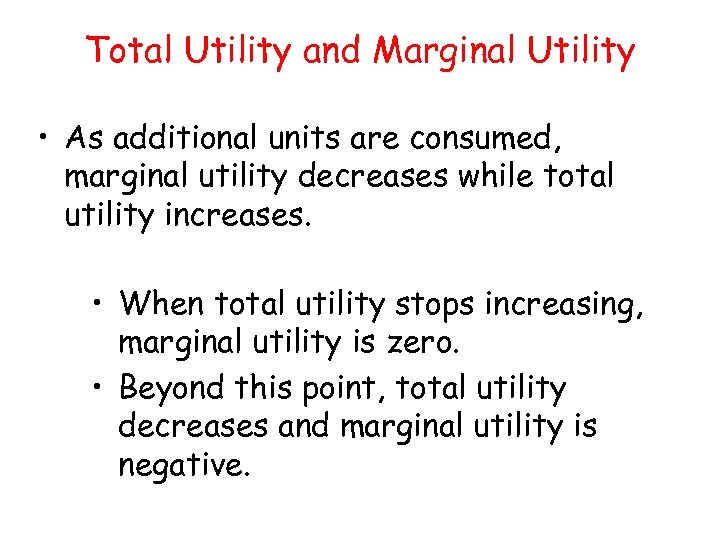Total Utility and Marginal Utility • As additional units are consumed, marginal utility decreases while total utility increases. • When total utility stops increasing, marginal utility is zero. • Beyond this point, total utility decreases and marginal utility is negative.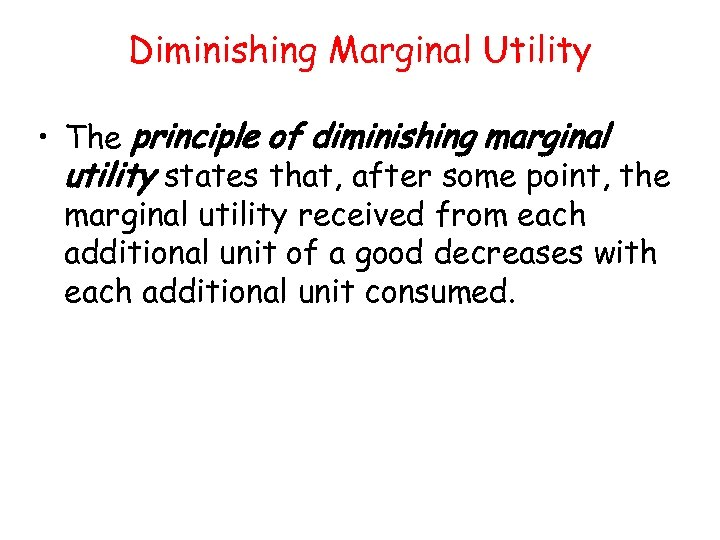Diminishing Marginal Utility • The principle of diminishing marginal utility states that, after some point, the marginal utility received from each additional unit of a good decreases with each additional unit consumed.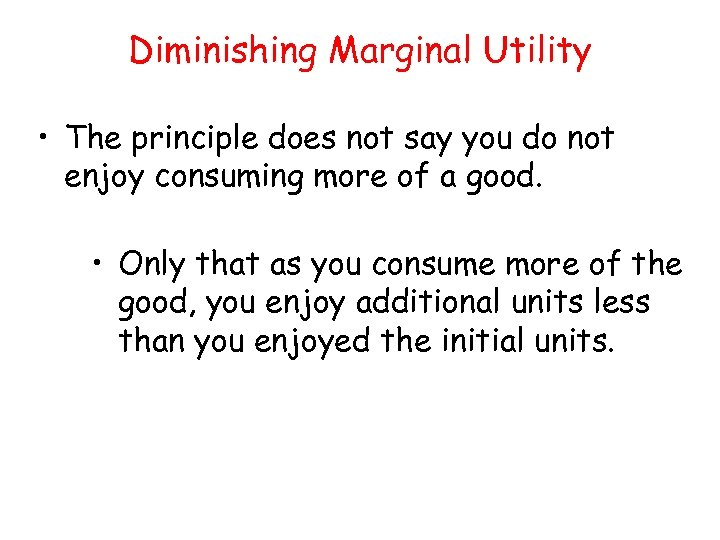Diminishing Marginal Utility • The principle does not say you do not enjoy consuming more of a good. • Only that as you consume more of the good, you enjoy additional units less than you enjoyed the initial units.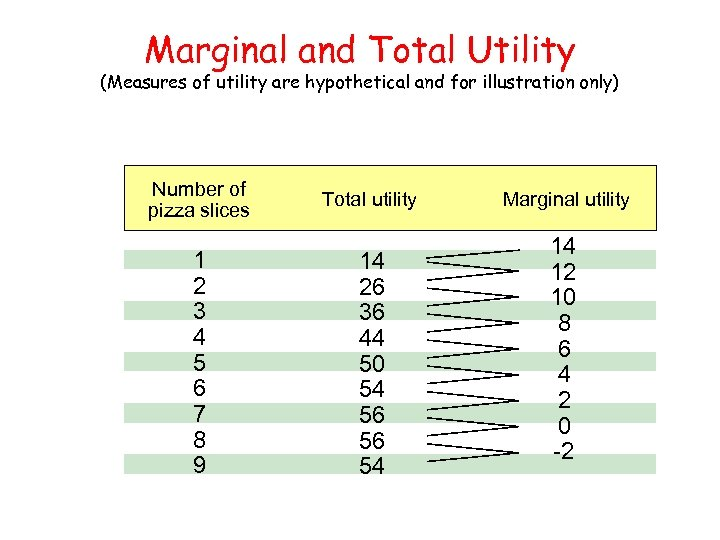Marginal and Total Utility (Measures of utility are hypothetical and for illustration only) Number of pizza slices 1 2 3 4 5 6 7 8 9 Total utility 14 26 36 44 50 54 56 56 54 Marginal utility 14 12 10 8 6 4 2 0 -2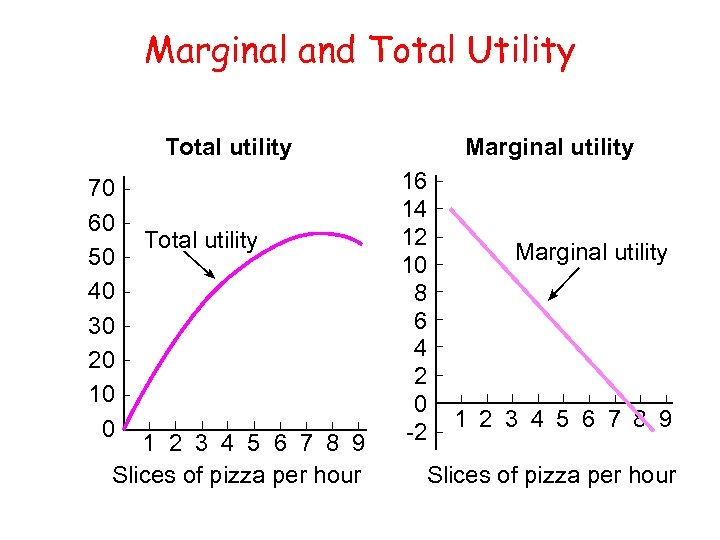Marginal and Total Utility Total utility 70 60 50 40 30 20 10 0 Total utility 1 2 3 4 5 6 7 8 9 Slices of pizza per hour Marginal utility 16 14 12 10 8 6 4 2 0 -2 Marginal utility 1 2 3 4 5 6 7 8 9 Slices of pizza per hour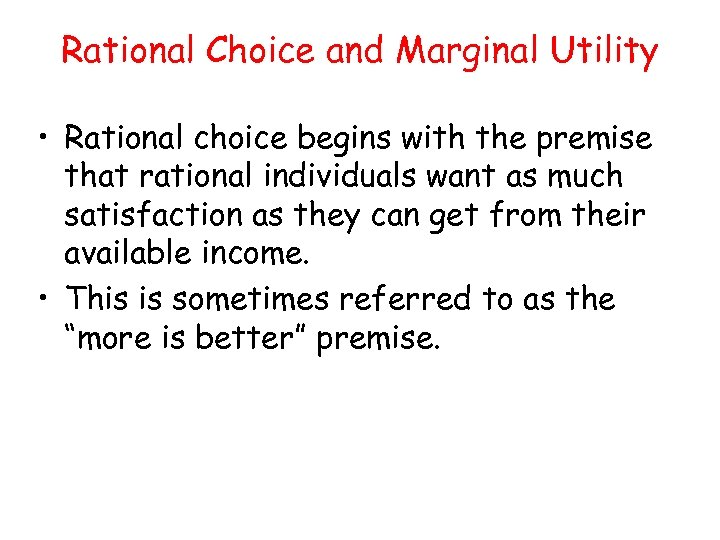Rational Choice and Marginal Utility • Rational choice begins with the premise that rational individuals want as much satisfaction as they can get from their available income. • This is sometimes referred to as the “more is better” premise.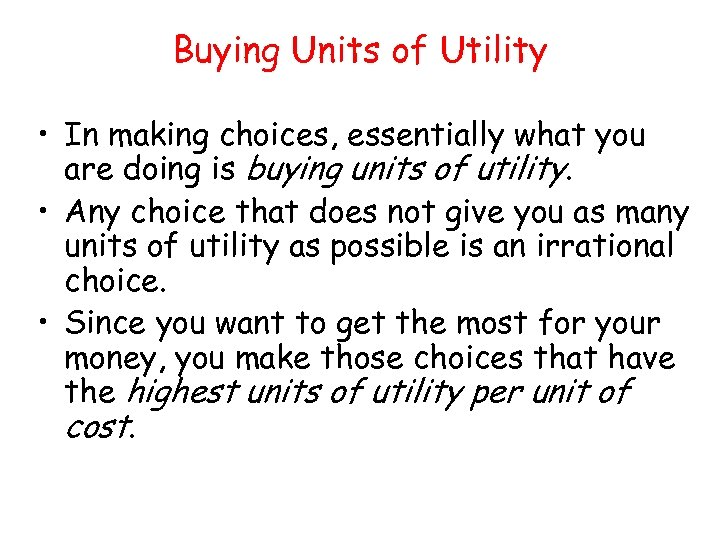Buying Units of Utility • In making choices, essentially what you are doing is buying units of utility. • Any choice that does not give you as many units of utility as possible is an irrational choice. • Since you want to get the most for your money, you make those choices that have the highest units of utility per unit of cost.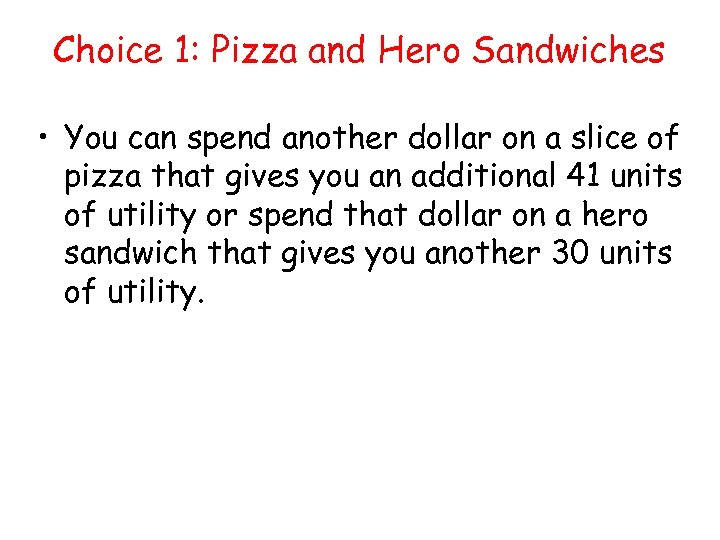Choice 1: Pizza and Hero Sandwiches • You can spend another dollar on a slice of pizza that gives you an additional 41 units of utility or spend that dollar on a hero sandwich that gives you another 30 units of utility.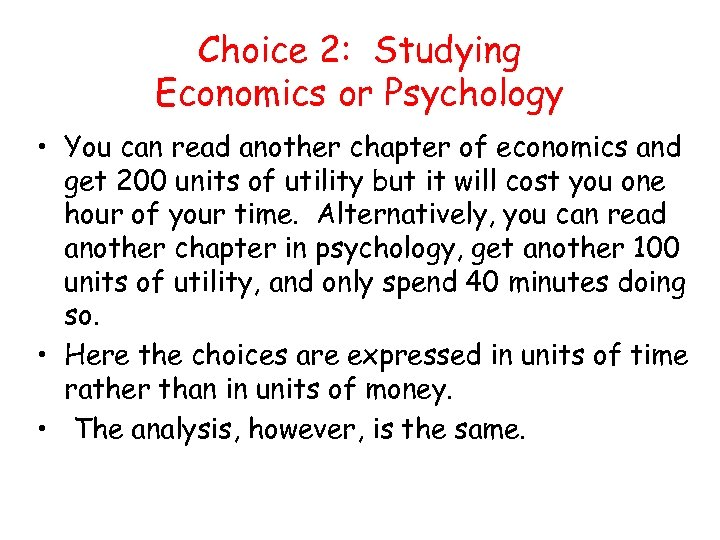Choice 2: Studying Economics or Psychology • You can read another chapter of economics and get 200 units of utility but it will cost you one hour of your time. Alternatively, you can read another chapter in psychology, get another 100 units of utility, and only spend 40 minutes doing so. • Here the choices are expressed in units of time rather than in units of money. • The analysis, however, is the same.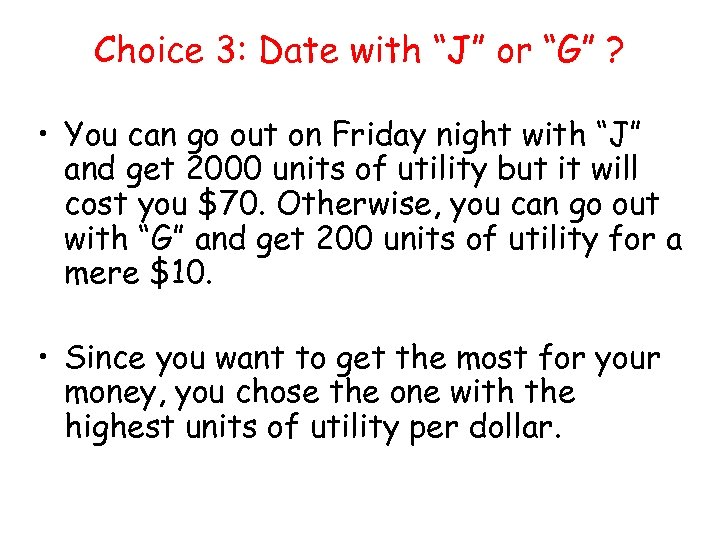Choice 3: Date with “J” or “G” ? • You can go out on Friday night with “J” and get 2000 units of utility but it will cost you \$70. Otherwise, you can go out with “G” and get 200 units of utility for a mere \$10. • Since you want to get the most for your money, you chose the one with the highest units of utility per dollar.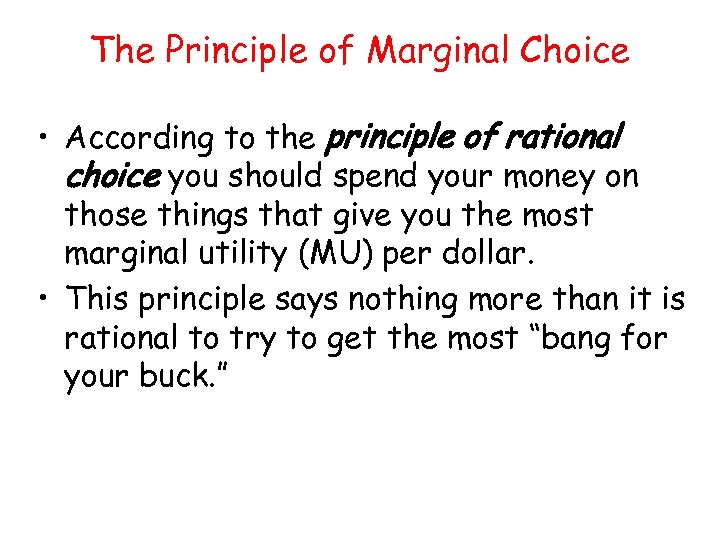The Principle of Marginal Choice • According to the principle of rational choice you should spend your money on those things that give you the most marginal utility (MU) per dollar. • This principle says nothing more than it is rational to try to get the most “bang for your buck. ”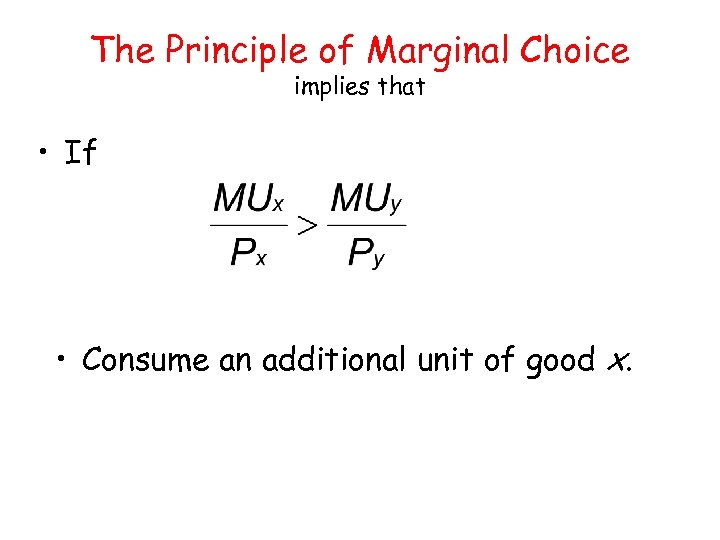The Principle of Marginal Choice implies that • If • Consume an additional unit of good x.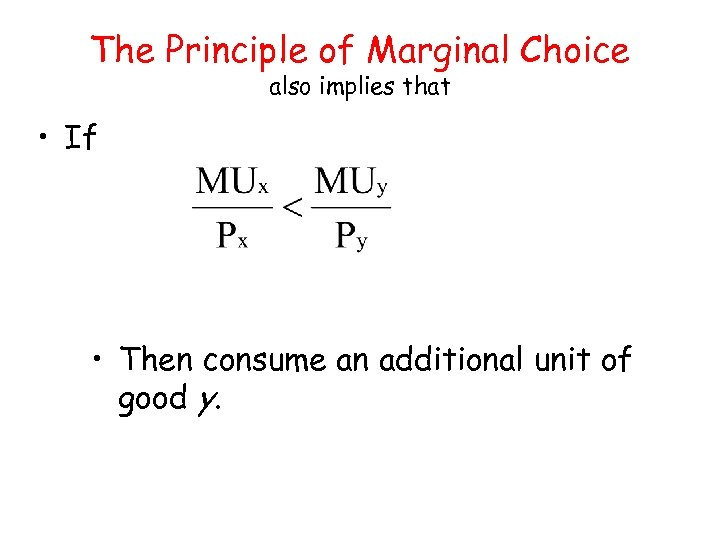The Principle of Marginal Choice also implies that • If • Then consume an additional unit of good y.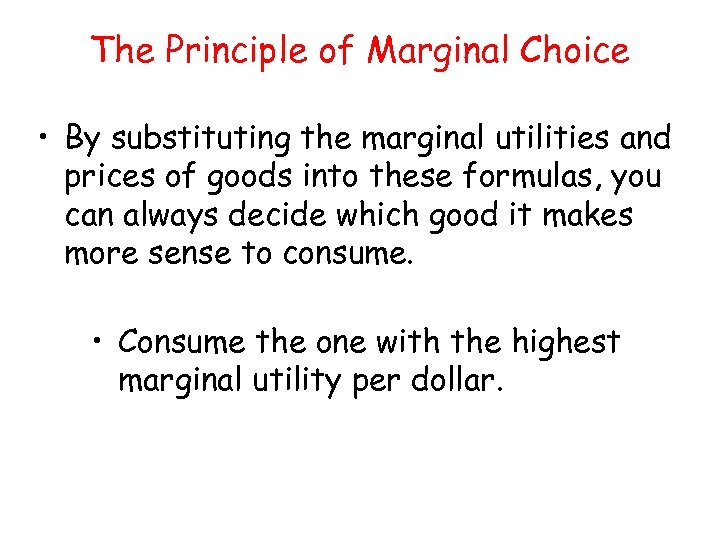The Principle of Marginal Choice • By substituting the marginal utilities and prices of goods into these formulas, you can always decide which good it makes more sense to consume. • Consume the one with the highest marginal utility per dollar.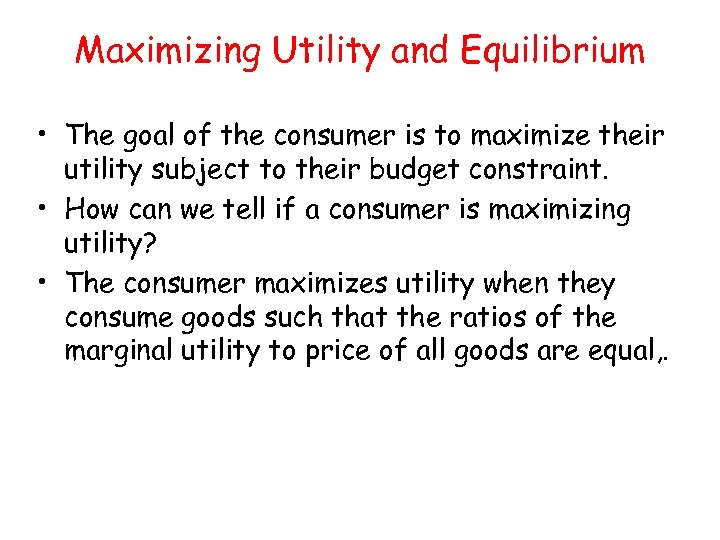Maximizing Utility and Equilibrium • The goal of the consumer is to maximize their utility subject to their budget constraint. • How can we tell if a consumer is maximizing utility? • The consumer maximizes utility when they consume goods such that the ratios of the marginal utility to price of all goods are equal, .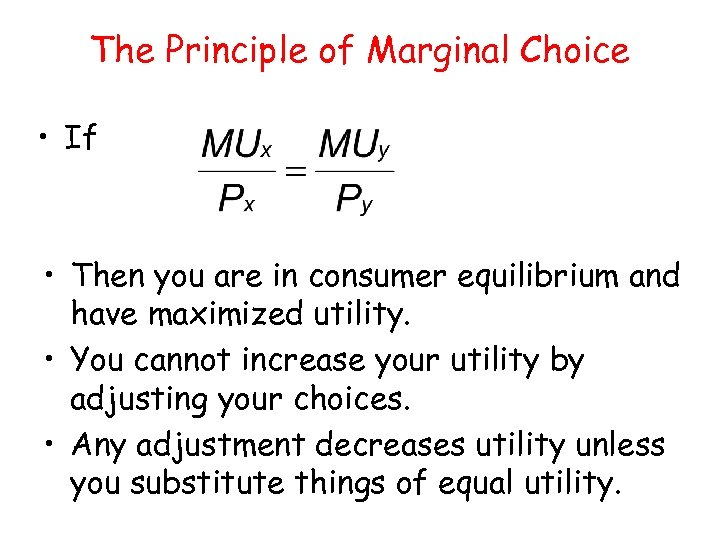The Principle of Marginal Choice • If • Then you are in consumer equilibrium and have maximized utility. • You cannot increase your utility by adjusting your choices. • Any adjustment decreases utility unless you substitute things of equal utility.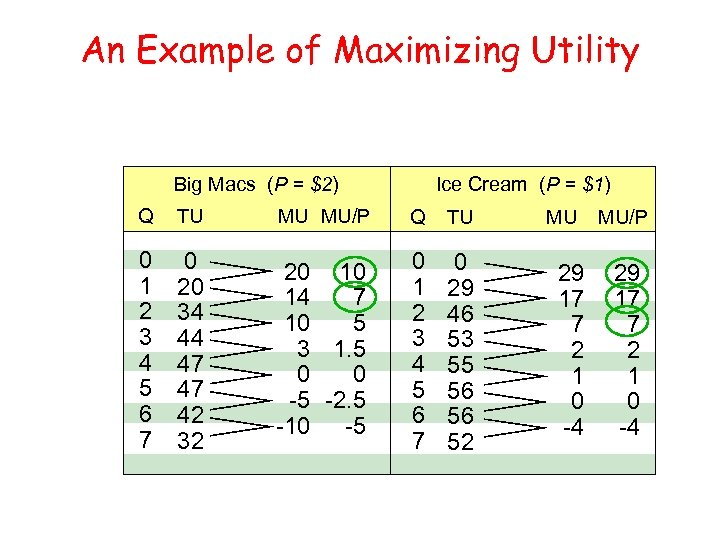An Example of Maximizing Utility Big Macs (P = \$2) Ice Cream (P = \$1) Q TU MU MU/P Q TU 0 1 2 3 4 5 6 7 0 20 34 44 47 47 42 32 20 10 14 7 10 5 3 1. 5 0 0 -5 -2. 5 -10 -5 0 1 2 3 4 5 6 7 0 29 46 53 55 56 56 52 MU 29 17 7 2 1 0 -4 MU/P 29 17 7 2 1 0 -4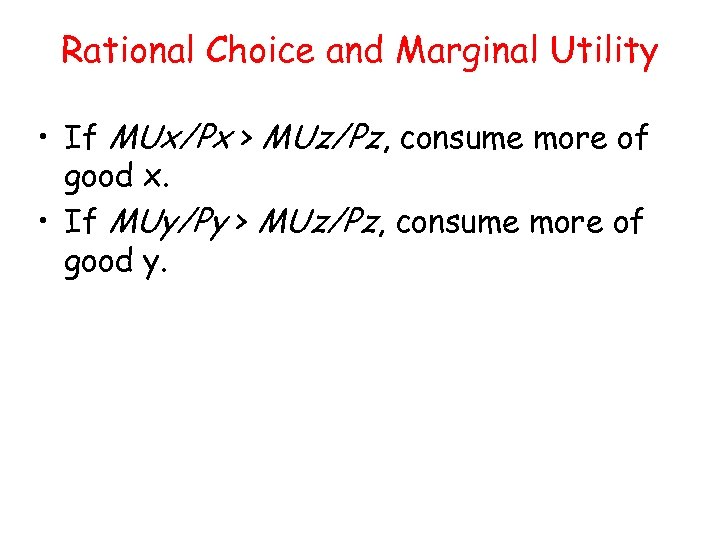Rational Choice and Marginal Utility • If MUx/Px > MUz/Pz, consume more of good x. • If MUy/Py > MUz/Pz, consume more of good y.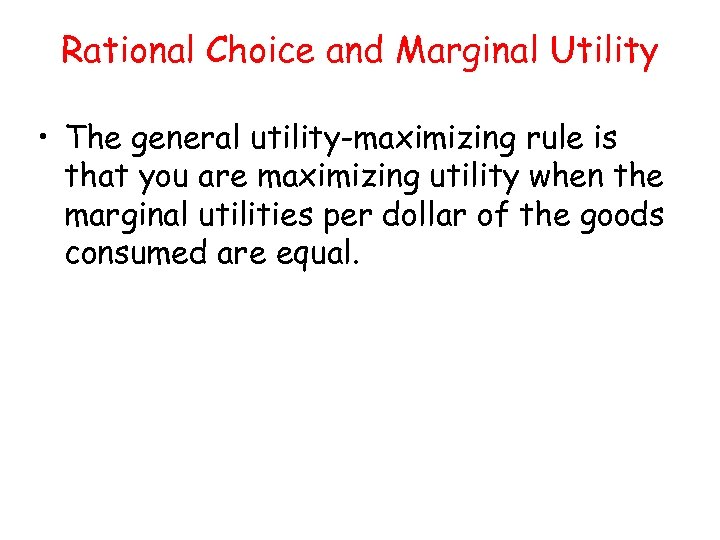Rational Choice and Marginal Utility • The general utility-maximizing rule is that you are maximizing utility when the marginal utilities per dollar of the goods consumed are equal.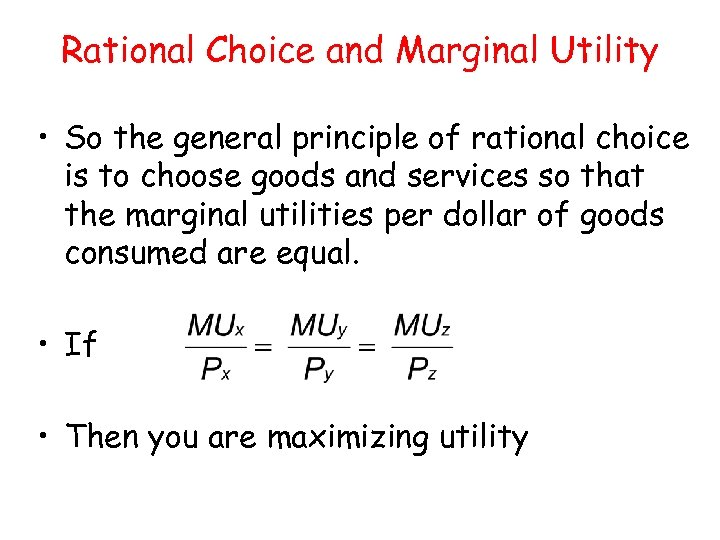Rational Choice and Marginal Utility • So the general principle of rational choice is to choose goods and services so that the marginal utilities per dollar of goods consumed are equal. • If • Then you are maximizing utility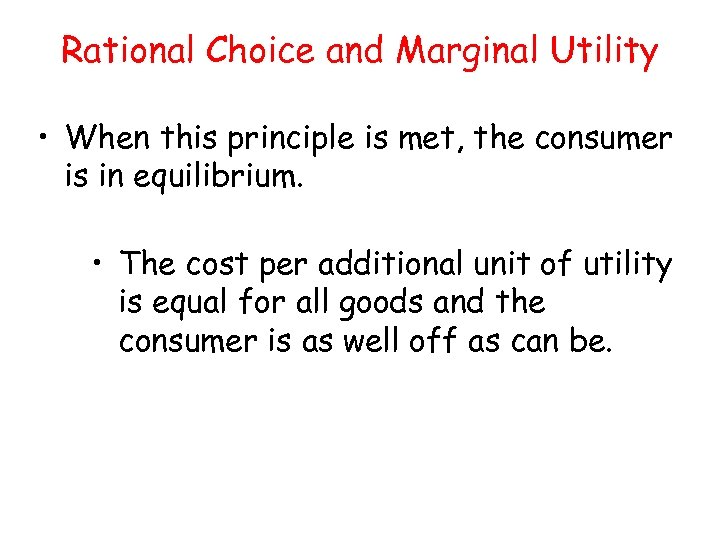Rational Choice and Marginal Utility • When this principle is met, the consumer is in equilibrium. • The cost per additional unit of utility is equal for all goods and the consumer is as well off as can be.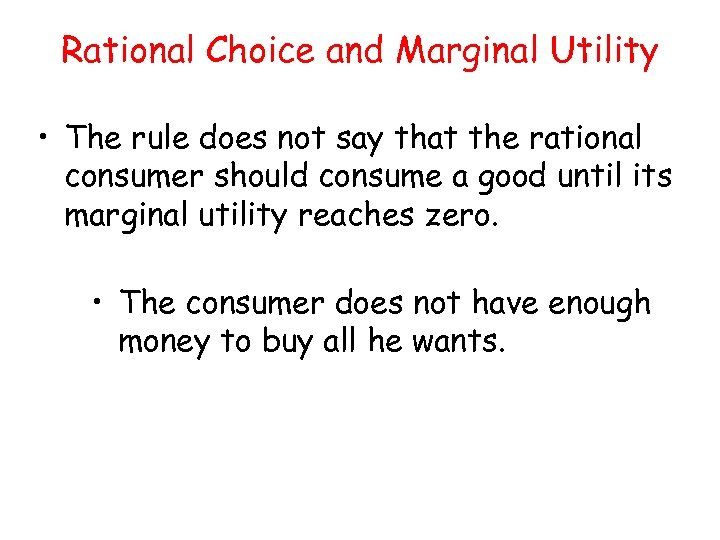Rational Choice and Marginal Utility • The rule does not say that the rational consumer should consume a good until its marginal utility reaches zero. • The consumer does not have enough money to buy all he wants.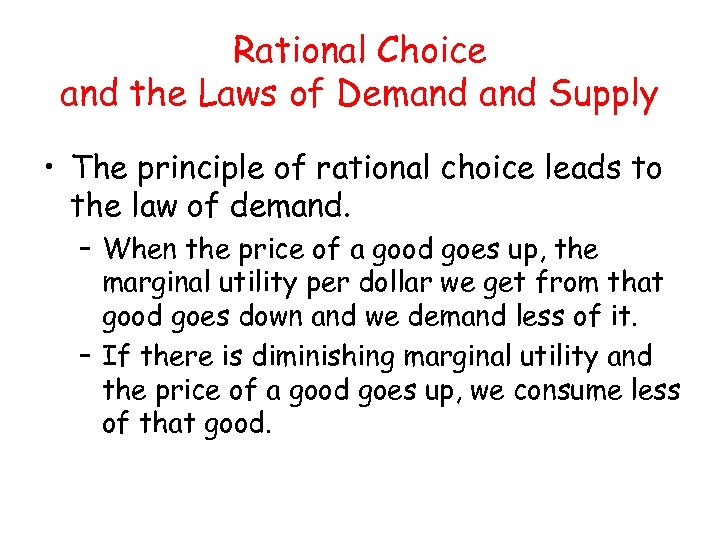Rational Choice and the Laws of Demand Supply • The principle of rational choice leads to the law of demand. – When the price of a good goes up, the marginal utility per dollar we get from that good goes down and we demand less of it. – If there is diminishing marginal utility and the price of a good goes up, we consume less of that good.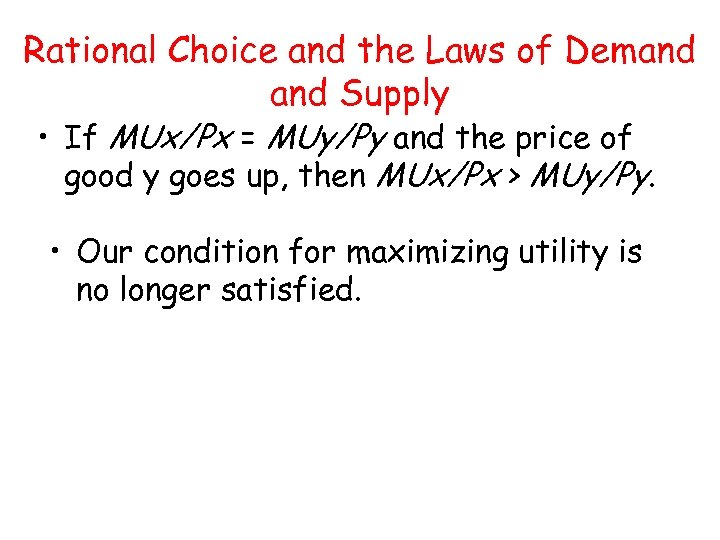Rational Choice and the Laws of Demand Supply • If MUx/Px = MUy/Py and the price of good y goes up, then MUx/Px > MUy/Py. • Our condition for maximizing utility is no longer satisfied.Rational Choice and the Laws of Demand Supply • To continue maximizing utility, we must somehow raise the marginal utility we get from the good whose relative price has risen and lower the marginal utility we get from a good whose relative price has fallen.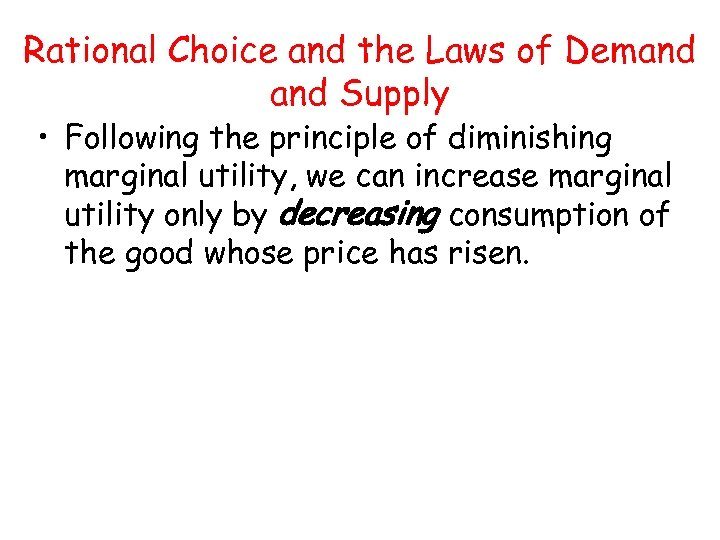Rational Choice and the Laws of Demand Supply • Following the principle of diminishing marginal utility, we can increase marginal utility only by decreasing consumption of the good whose price has risen.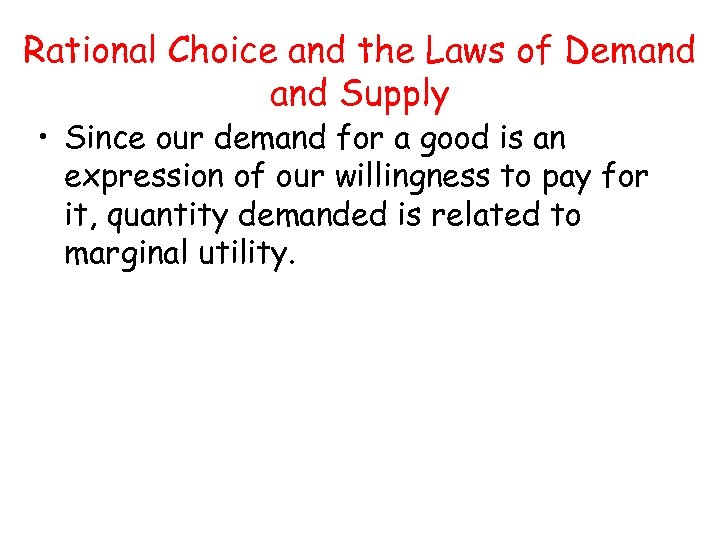Rational Choice and the Laws of Demand Supply • Since our demand for a good is an expression of our willingness to pay for it, quantity demanded is related to marginal utility.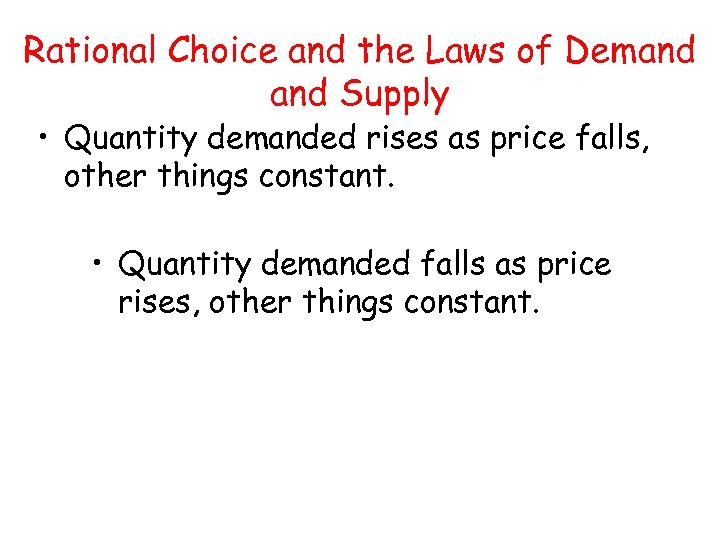Rational Choice and the Laws of Demand Supply • Quantity demanded rises as price falls, other things constant. • Quantity demanded falls as price rises, other things constant.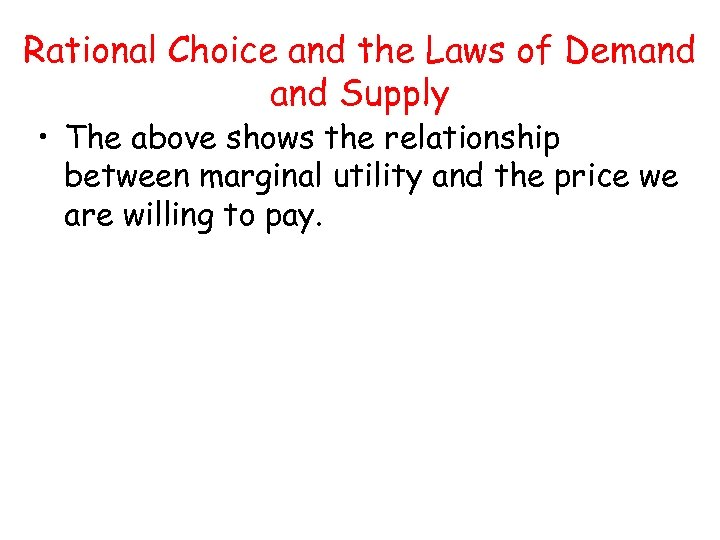Rational Choice and the Laws of Demand Supply • The above shows the relationship between marginal utility and the price we are willing to pay.Rational Choice and the Laws of Demand Supply • Since our demand for a good is an expression of our willingness to pay for it, quantity demanded is related to marginal utility.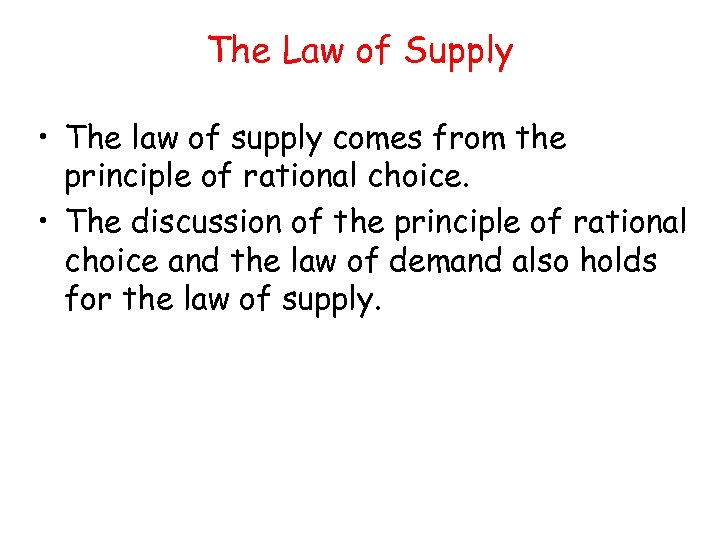The Law of Supply • The law of supply comes from the principle of rational choice. • The discussion of the principle of rational choice and the law of demand also holds for the law of supply.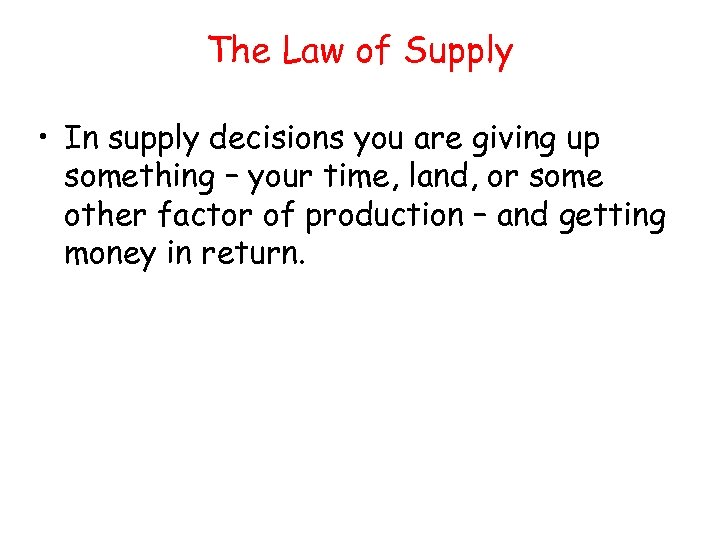The Law of Supply • In supply decisions you are giving up something – your time, land, or some other factor of production – and getting money in return.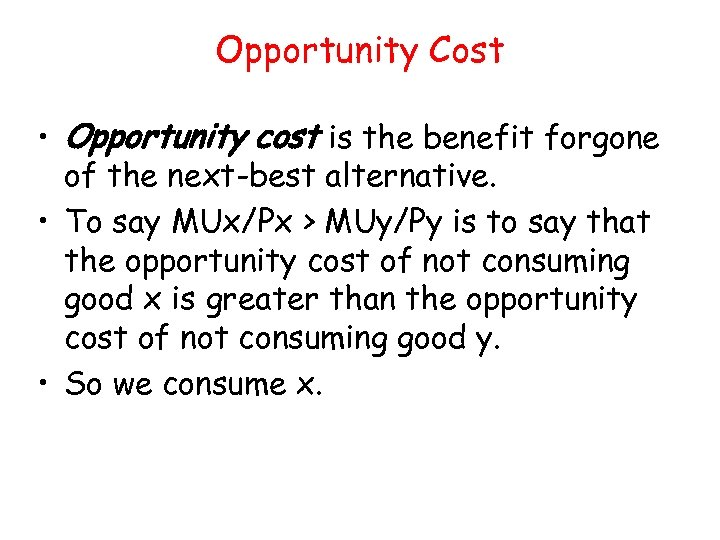Opportunity Cost • Opportunity cost is the benefit forgone of the next-best alternative. • To say MUx/Px > MUy/Py is to say that the opportunity cost of not consuming good x is greater than the opportunity cost of not consuming good y. • So we consume x.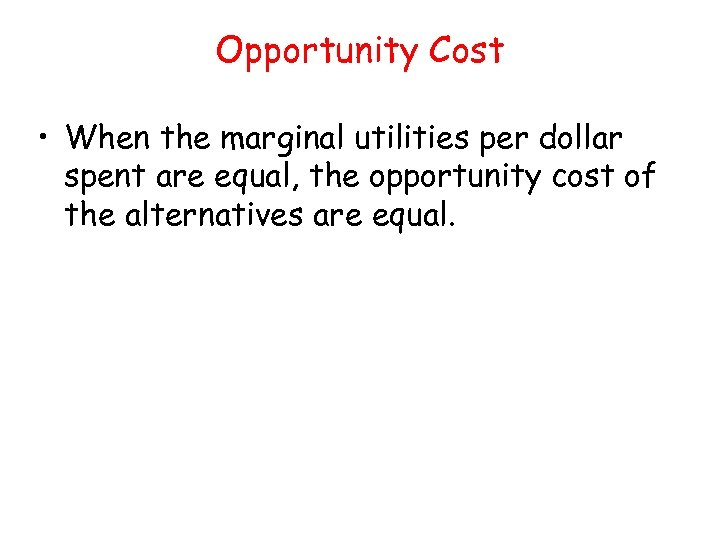Opportunity Cost • When the marginal utilities per dollar spent are equal, the opportunity cost of the alternatives are equal.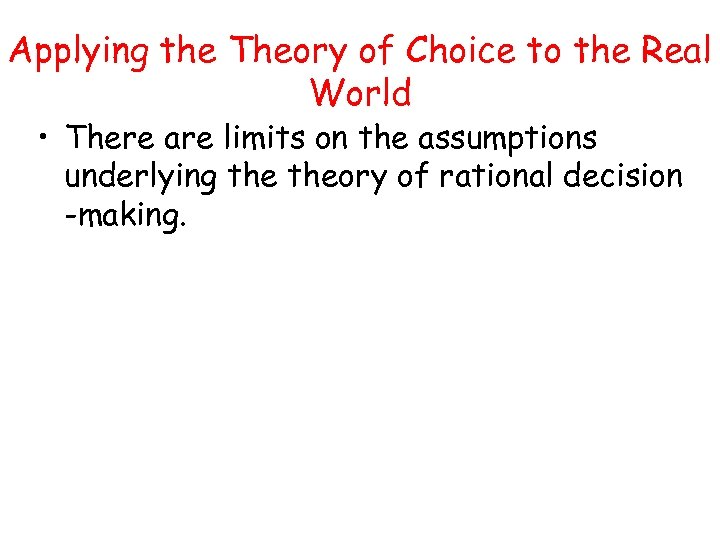Applying the Theory of Choice to the Real World • There are limits on the assumptions underlying theory of rational decision -making.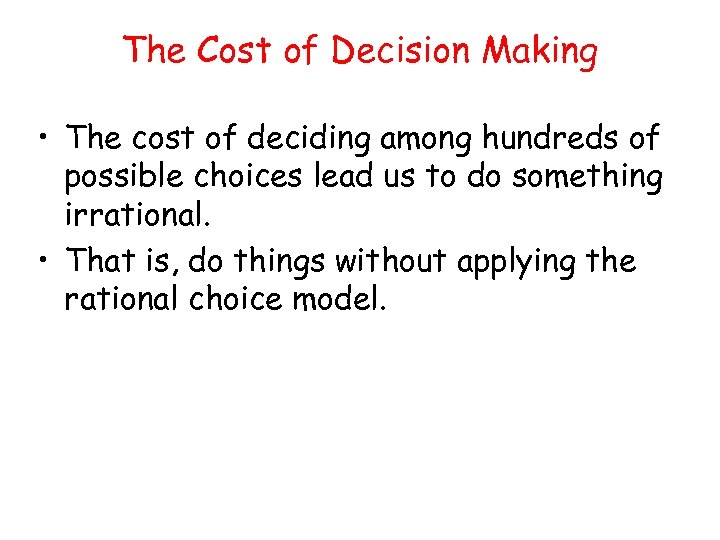The Cost of Decision Making • The cost of deciding among hundreds of possible choices lead us to do something irrational. • That is, do things without applying the rational choice model.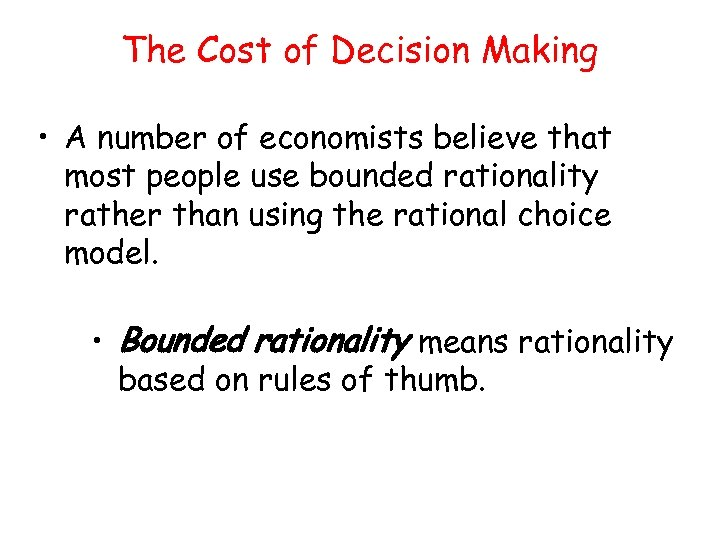The Cost of Decision Making • A number of economists believe that most people use bounded rationality rather than using the rational choice model. • Bounded rationality means rationality based on rules of thumb.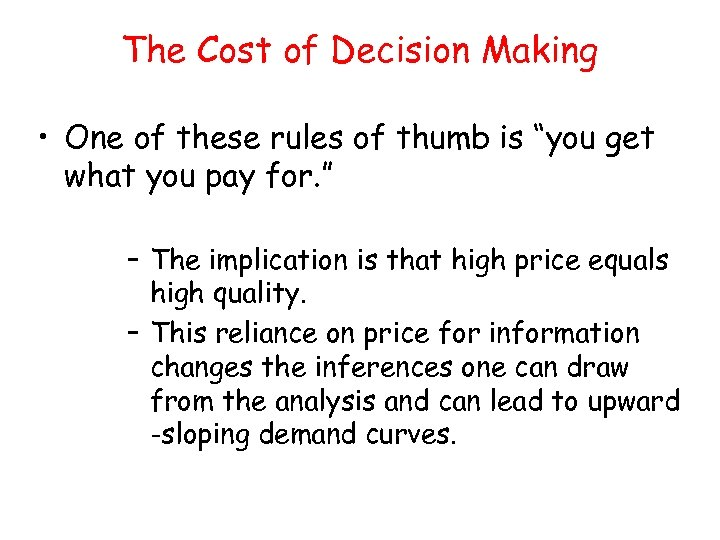The Cost of Decision Making • One of these rules of thumb is “you get what you pay for. ” – The implication is that high price equals high quality. – This reliance on price for information changes the inferences one can draw from the analysis and can lead to upward -sloping demand curves.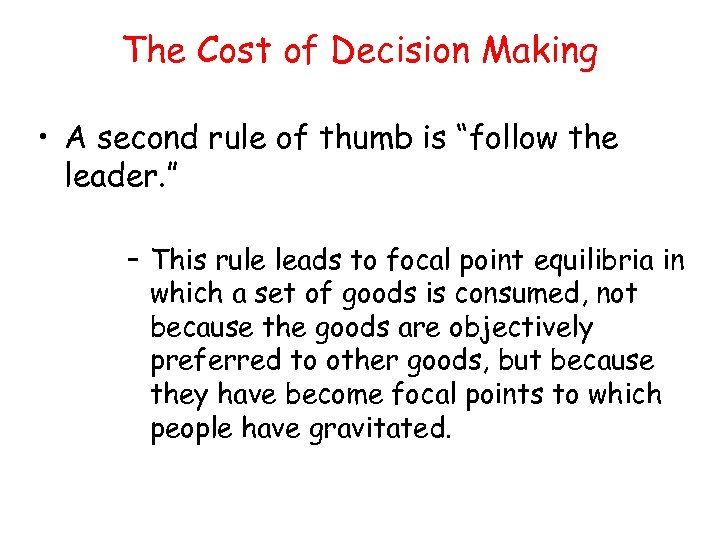The Cost of Decision Making • A second rule of thumb is “follow the leader. ” – This rule leads to focal point equilibria in which a set of goods is consumed, not because the goods are objectively preferred to other goods, but because they have become focal points to which people have gravitated.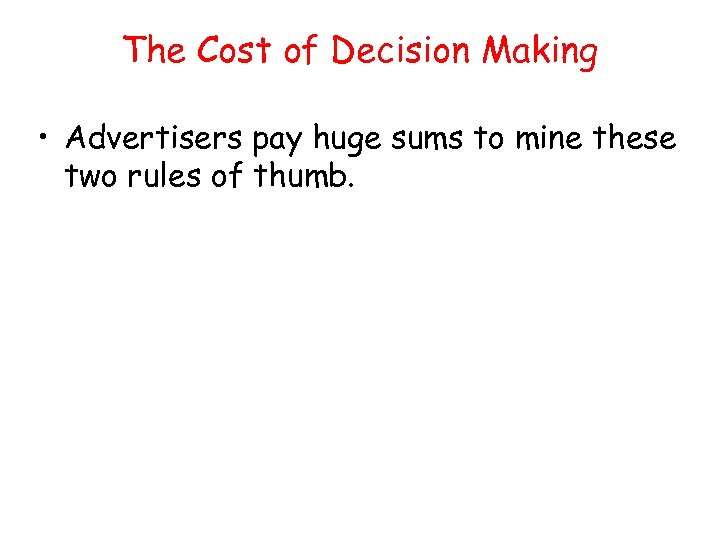The Cost of Decision Making • Advertisers pay huge sums to mine these two rules of thumb.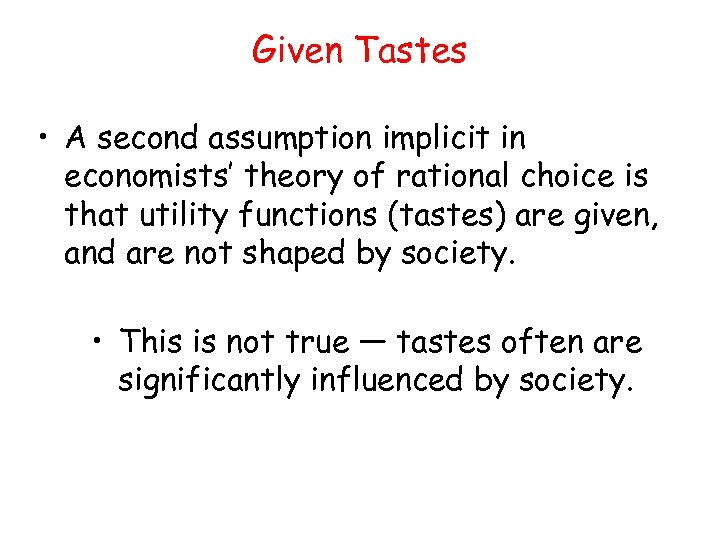Given Tastes • A second assumption implicit in economists’ theory of rational choice is that utility functions (tastes) are given, and are not shaped by society. • This is not true — tastes often are significantly influenced by society.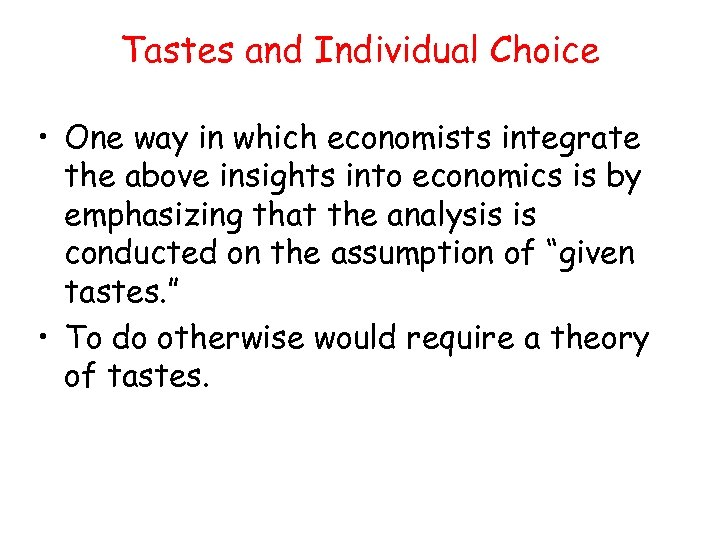Tastes and Individual Choice • One way in which economists integrate the above insights into economics is by emphasizing that the analysis is conducted on the assumption of “given tastes. ” • To do otherwise would require a theory of tastes.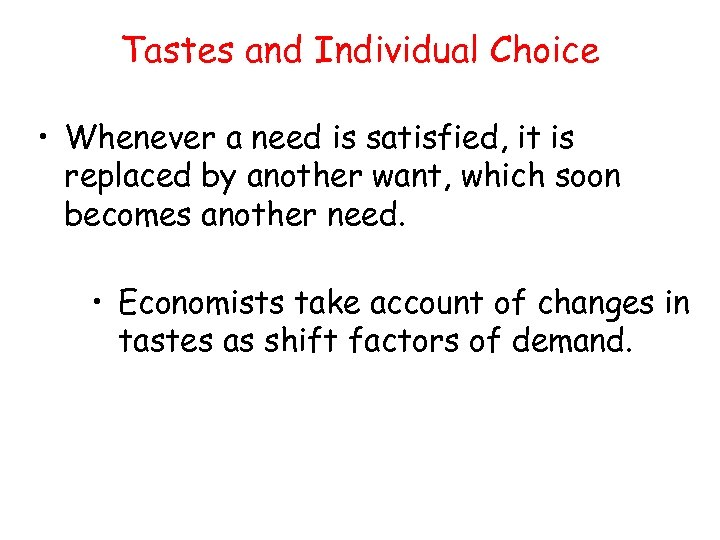Tastes and Individual Choice • Whenever a need is satisfied, it is replaced by another want, which soon becomes another need. • Economists take account of changes in tastes as shift factors of demand.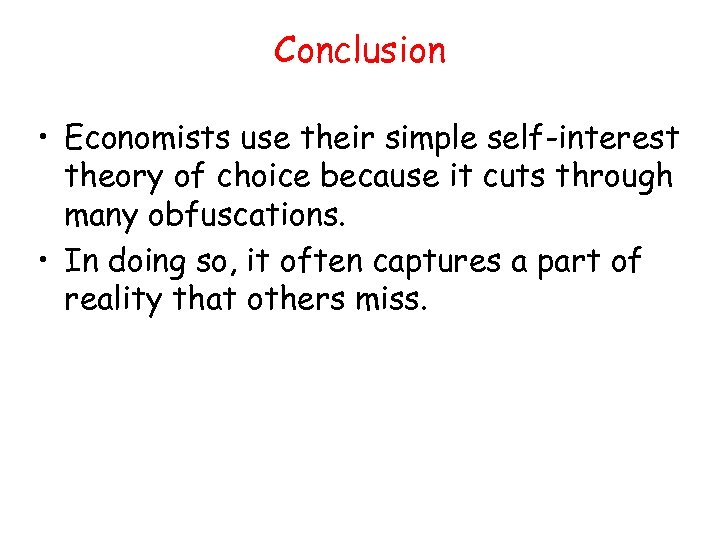Conclusion • Economists use their simple self-interest theory of choice because it cuts through many obfuscations. • In doing so, it often captures a part of reality that others miss.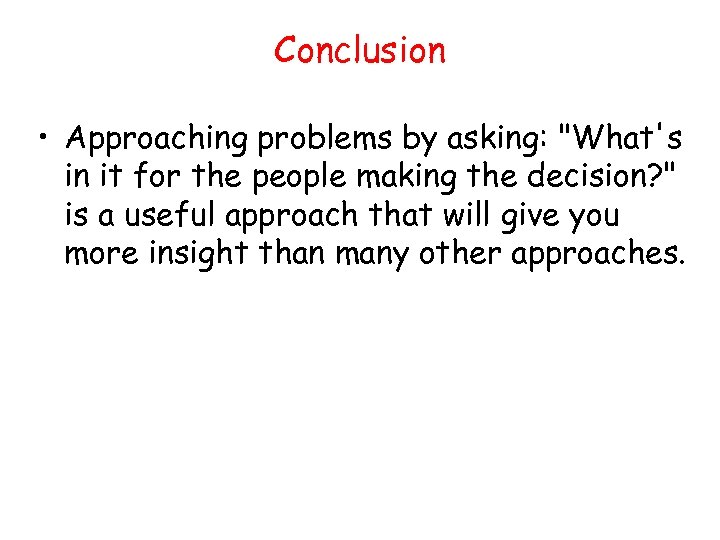Conclusion • Approaching problems by asking: "What's in it for the people making the decision? " is a useful approach that will give you more insight than many other approaches.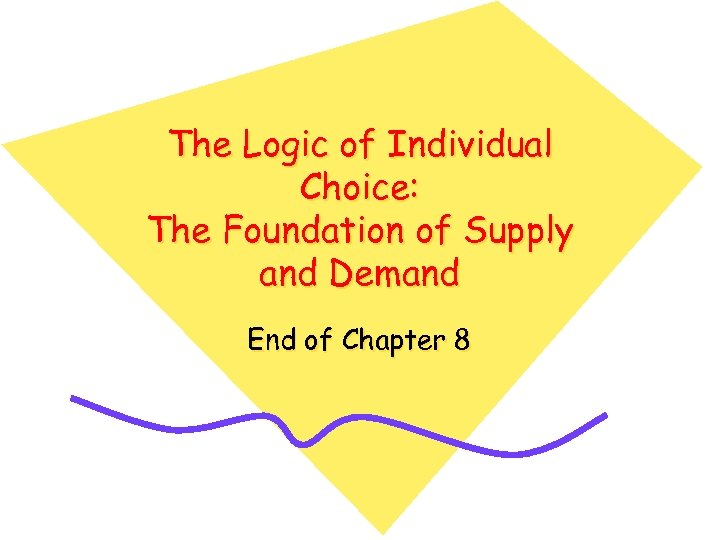The Logic of Individual Choice: The Foundation of Supply and Demand End of Chapter 8